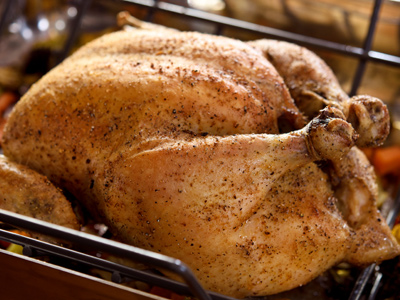Chicken should be cooked for 50 minutes for every kg. It will take 150 minutes to cook a 3kg chicken.

# Ratio (Year 5)

In Year 5, the third year in KS2 Maths, you'll learn a cool thing! It's about comparing numbers using ratios. Imagine numbers on either side of a colon, like 2:1 or 3:1 - that's a ratio. It's like saying how things relate to each other.

For example, in a bowl of fruit, there are 8 apples and 6 pears. The ratio of apples to pears is 8:6 (or 4:3, which is the same). Turn it around, and it's the ratio of pears to apples, 6:8 (or 3:4). Ratios help us understand how amounts compare!

Want to be a ratio expert? Take our fun quiz to find out more about ratios in KS2 Maths!

1.
The ratio between blue boxes and yellow boxes is 3:2. If there are 4 yellow boxes how many blue boxes are there?
2
3
6
10
The amount of yellow boxes has been multiplied by 2 so we must multiply the blue boxes by 2
2.
If there is a 5:2 ratio between black squares and white squares which number represents the white squares?
1
2
5
10
The 2 represents the white squares while the 5 represents the black squares as black was mentioned first
3.
Chicken should be cooked for 50 minutes for every kg. How long will it take to cook a 3kg chicken?
100 minutes
150 minutes
175 minutes
200 minutes
Or two and a half hours
4.
When making a cake, for every 400g of flour 200g of sugar is required. What is another way of saying this?
We require half as much flour as sugar
We require twice more sugar than flour
We require the same amount of sugar and flour
We require half as much sugar as flour
The ratio between flour and sugar is 2:1
5.
Zara uses 20ml of fruit concentrate for every 60ml of water. How much fruit concentrate will she require for 180ml of water?
20ml
40ml
50ml
60ml
The amount of water has increased 3 times so the same must happen to the amount of fruit concentrate
6.
John has twice as many stamps than Yasif. If Yasif has 5 stamps how many stamps does John have?
5
10
15
20
The ratio between John and Yashif's stamps is 2:1
7.
Lucy mixes 4 small pots of white paint with 1 small pot of red paint to make 1 large tin of pink paint. She requires 5 large tins of pink paint. How many small pots of white paint does she need?
5 tins
10 tins
20 tins
30 tins
There are 4 pots of white paint in each tin of pink, so 5 tins of pink means 4 x 5 = 20
8.
In every 10 bricks, 3 are red. We have 30 bricks so how many are red?
3
6
9
15
The number of bricks has been multiplied by 3 so the number of red bricks needs to be multiplied by 3
9.
For every 2 black squares there is 1 white square. What is the ratio of black to white?
2:1
1:2
3:2
1:1
It there is a ratio of 2:1 then there is twice as much of the first thing than the last
10.
There are 3 yellow boxes for every 1 blue box. If there are 6 yellow boxes how many blue boxes are there?
2
3
6
12
If there are 6 yellow boxes then that is twice the number given in the ratio. Therefore we must multiply the number of blue boxes by 2